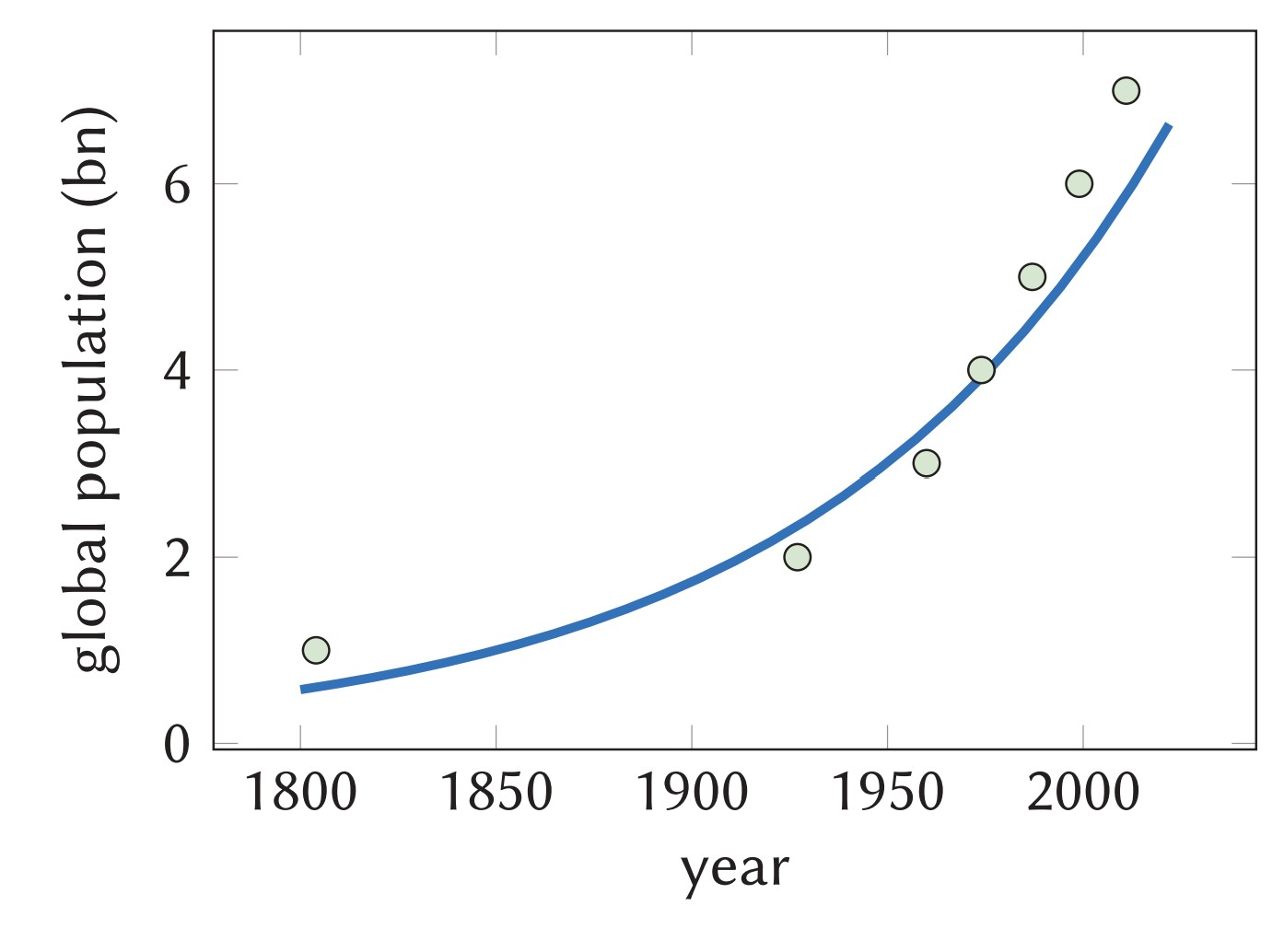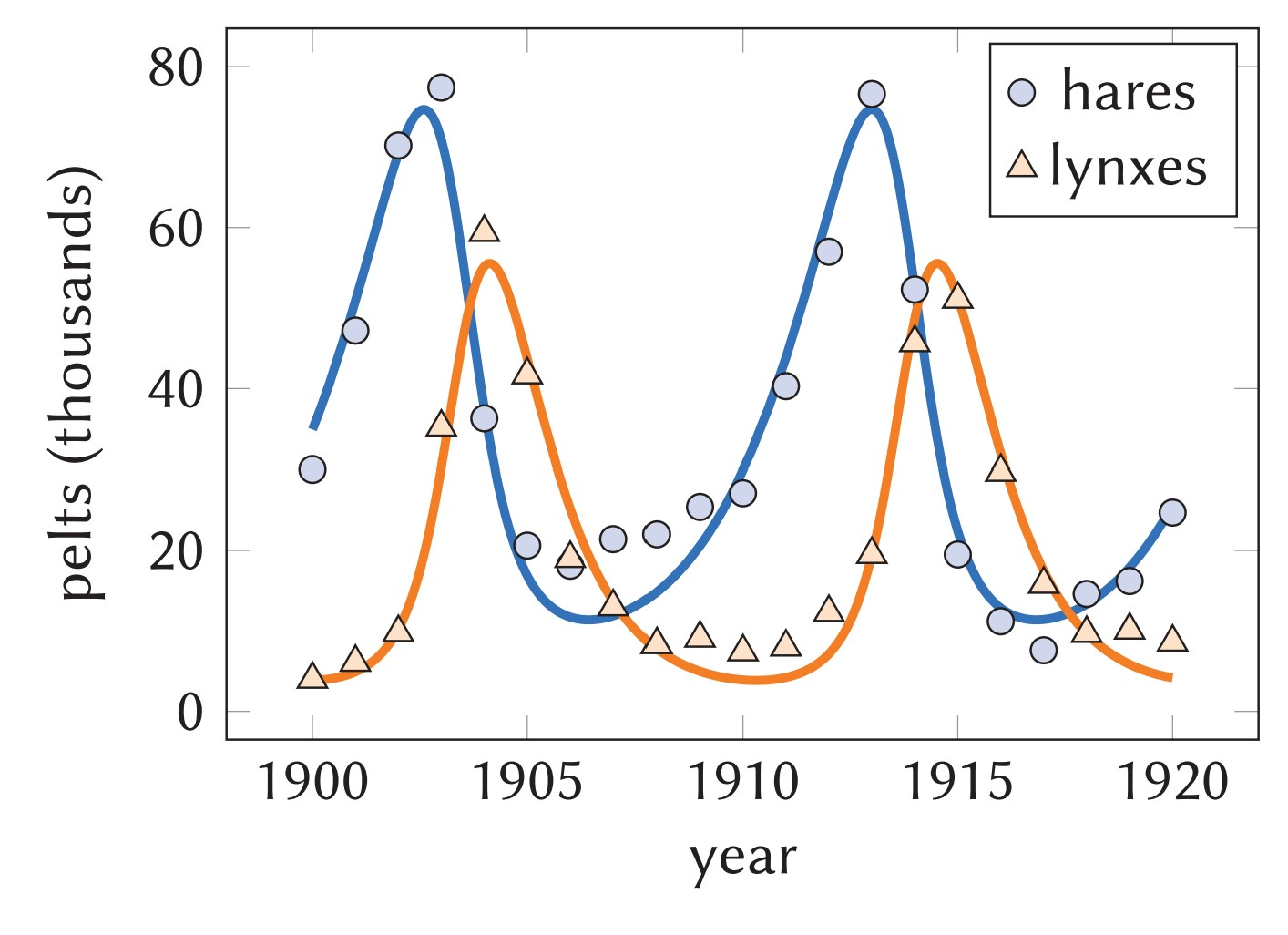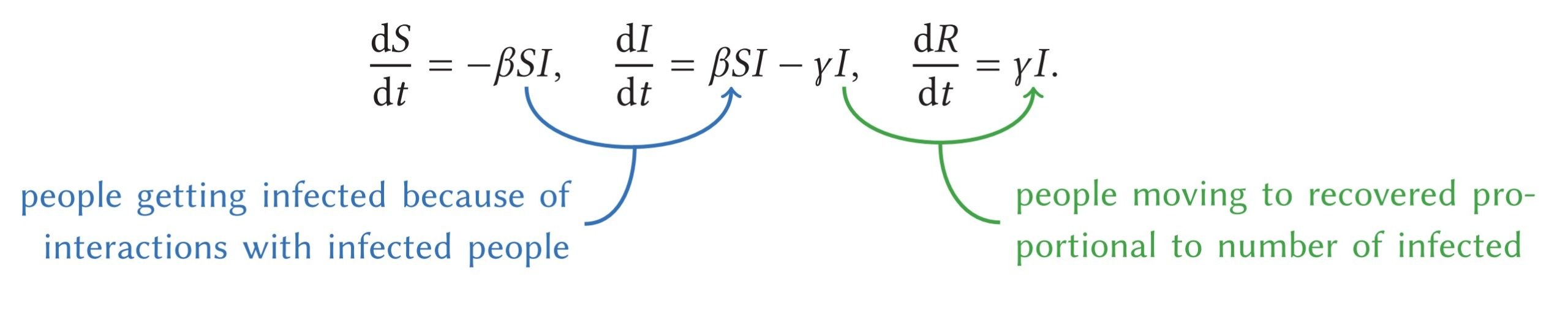# Celebrating simple mathematical models

Hollis Williams explores the power even simple models can have in describing the world around us.In their most uncomplicated form mathematical models are essentially just mathematical descriptions of real-world systems. Stringing together variables and parameters into an equation we can attempt to describe complex behaviours that change with respect to time.

Today mathematical models are used for everything, from predicting exam grades, to the Earth’s climate a hundred years from now. But mathematical models don’t need to be complicated to be useful. Even simple models have the power to reveal insights about a problem, to guide us in the decisions we make and to reveal unexpected consequences of our actions. They even stand up surprisingly well to more sophisticated models, still managing to capture subtle dynamics, with far less computational expense.

I think simple mathematical models are worth celebrating, so here I’m going to discuss three simple but very important models. You might be used to thinking of physics or engineering as the typical subjects in which mathematical models are employed, so let’s turn this on its head and describe some models from sociology and biology.

## Survival of the fittest

A key question in sociology and economics is how the size of human populations change over time, and when we talk about something changing with respect to time we use a differential equation to describe it.

The simplest model of population growth comes from 1798: the Malthusian model, $\frac{\mathrm{d}P}{\mathrm{d}t}=\alpha P,$ where $P(t)$ is the size of the population at time $t$, and $\alpha$ is the constant growth rate of the population. This model argues that the rate at which the population changes over time depends linearly on $P$, the number of people currently able to reproduce or die. If we use the initial condition, that at our commencement point in time the population size is $P(0)=P_0$, then this differential equation can be solved to give $P(t)=P_0 \mathrm{e}^{\alpha t}.$This is a very simple conclusion, but is the model any good?

The graph on the right shows how global human population has changed over the last 220 years. The green circles represent the real numbers: data from the United Nations, and the blue line is the mathematical model’s prediction $P(t)$, where $\alpha=0.011$ and $P_0$ is chosen so $P(1850)$ is 1 billion people.

This is a fairly good match; both prediction and data show exponential growth. But what about the future? In Factfulness, the physician and academic Hans Rosling discusses how as countries get richer, citizens tend to have fewer children and growth rate slows, so $\alpha$, and the slope of our graph, should decrease over time.

So is the model perfect? No, but one simple equation, whose solution can be solved used a pen and a single side of A4 paper does a pretty good job of describing how human populations have changed for the last few centuries.

## The lynx effect

Now let’s look at a slightly more complicated mathematical model, how the populations of two different animal species interact.

The Canada lynx is a wild cat that lives broadly in Canada and Alaska, with a diet consisting mostly of the snowshoe hare, which is native to the same geographical region. Let’s try to model how the numbers of each animal changes over time.

We use variable $P$ to represent the hare population, and $Q$ for the lynx. The Lotka-Volterra model, from the early 20th century, for how the sizes of these populations change is given below:We can adjust the positive constants, $\alpha$, $\beta$, $\gamma$ and $\delta$, to fit real-life measurements (why shouldn’t we necessarily want $\beta=\delta$?): in modelling we call these parameters.

Unlike the Malthusian model, solving this system of equations has to be done numerically. The solution is periodic, both the predator and prey populations oscillate, with the predators population trailing slightly behind that of its prey.

Once more, we can compare our model to reality. There is plenty of data recording lynx and hare populations, or to be specific, there is plenty of real historic data about how many lynx and hare pelts were collected by fur traders in the area.

The plot below shows the comparison between the solution of the system and pelt counts from between 1900 to 1920. I’ve found the best-fitting values for the parameters and the initial conditions by using a least squares method (if you want the details, Joseph Mahaffy’s lecture notes from San Diego State University take you through it).

Itmight not seem intuitive why these populations should oscillate, but let’s have a think. When there is an abundance of tasty hares, there’s more than enough food to go around and the population of the lynxes swells. But a large and greedy population rapidly depletes the hare stash, and food shortages mean a large population can’t be supported for long, and the number of lynxes falls.

Not bad for a couple of differential equations: the model isn’t too hard to understand, it describes this common predator-prey situation well, and it reveals this interesting regular periodicity to how their population sizes change.

## Hot topic

Moving on to our final model, we turn our attention to some of the hottest maths in the news. It would be difficult to have missed talk about the ‘R number’ in news reports on the ongoing Covid-19 pandemic. This number, called $R_0$ in the mathematical literature, is the basic reproduction number. Loosely speaking, $R_0$ tells us how many people we expect one person to infect, and it is typically used to refer to the spread of a disease prior to any government interventions to reduce transmission.

$R_0$ comes from the SIR model for the spread of infectious diseases. The key variables in this model come from how we split the initially susceptible population of the country into three groups:

• $S(t)$, the number of people still susceptible to the disease,
• $I(t)$, the number of infected people, and
• $R(t)$, the number of people who have recovered from the disease and developed immunity.

If we make the key assumptions that the total initially susceptible population size does not change over time, $S+I+R=N$, and that the population is completely homogeneous, this then leads directly to a system of nonlinear ordinary differential equations,This is similar to Lotka–Volterra. There, $\delta xy$ represents the growth of the predator population since $xy$ represents interactions. What do you think $\beta SI$ represents here?

We have parameters for the infection rate, $\beta$, and the recovery rate, $\gamma$. The number $R_0$ is the rate at which secondary cases are produced, multiplied by the average infectious period, $R_0=\beta N \times \frac{1}{\gamma} = \frac{\beta N}{\gamma}.$

What happens in virus outbreak when no measures are made to contain it? Let’s look at the solution curves for $S$, $I$ and $R$. It’s possible to find these numerically if we choose some parameters. Fitting the model to data from the first wave of Covid-19 in Italy suggests a good match with $\beta N=0.180\,\mathrm{day}^{-1}$, $\gamma=0.037\,\mathrm{day}^{-1}$.

If we start with one infected person, $I=1$, and having everyone initially susceptible to start, $S(0)=N$, applying these parameters to the UK gives us plots for $S$, $I$ and $R$ below on the left.That’s a peak of over 120,000 infections, 100 days after the first infection. A pretty scary picture, and clear warning that interventions need to be made! If you’re not convinced, at the start of 2020, the UK had just 5,900 critical care beds. Making an assumption that one in ten of those infected in the first wave would need a critical care bed in hospital, then the model predicts that without intervention the NHS would be overwhelmed less than three months after patient zero contracted the disease.

Luckily, on a more positive note, this model also tells us what to do. We have a few different avenues to take action. We could try to change the number of people susceptible $S(t)$, by introducing a vaccine. Or we could try to decrease the average infectious period $1/\gamma$, but this is tricky if we don’t know much about the virus. Possibly the cheapest and easiest solution we could try is to tackle our parameter $\beta$. $\beta$ represents the infection rate; for $R_0$ to go down we need $\beta$ to be smaller. That means for every person who catches the disease we need them to interact with fewer others. The solution? Well perhaps we could consider quarantines, nationwide lockdowns, mask wearing or social distancing… do you see where we’re going here?

For the parameters we’ve chosen $R_0\approx4.9$. For England, with the initial strain in a fully susceptible population, the more sophisticated models from the team at Imperial College London (which were the models used to inform government policy), found $R_0$ at the time to be between 2.5 and 3.3.

But if we have more sophisticated models, why bother with the simple ones at all? Even with modern computing power, simple models are less computationally expensive. This SIR model runs instantaneously on my desktop computer with a few lines of code; the Imperial models use a huge amount of code which needs considerable time to run on a large computer cluster. Even this simple SIR model correctly predicts that $R_0>1$ and hence that the virus spreads, because each person infects more than one other person.

Furthermore, a simple model like this helps the public understand the epidemiological risks of a new virus. The solution curves are intuitive, and the figures arising from the model, like $R_0$, are comprehensible enough to be discussed on primetime news channels. It’s rare for mathematical terminology to seep into public discourse: it takes a very powerful, but simple model to do that.

## A happy conclusion

Simple mathematical models are a great gateway into understanding both mathematical concepts and the workings of systems the mathematics seeks to describe. Even very simple models can provide powerful insights; insights which can be gained from more complicated models but only at the expense of elegant equations and quick computations.

Yet there are plenty of real-world problems where a nice simple mathematical model is still lacking. If you’ve played with sand on a beach you’ll know that a collection of grains can flow like a liquid. In this area—known as granular flows—we are still lacking simple models for such a fluid which capture the observed behaviour. Why not consider your favourite physical system and see if you can come up with a simple mathematical model to describe it? Your model might just provide you with a new wealth of insight into what’s actually happening!Hollis is a PhD student at the University of Warwick who occasionally dabbles in mathematics. He is a mediocre but enthusiastic squash player.
+ More articles by Hollis